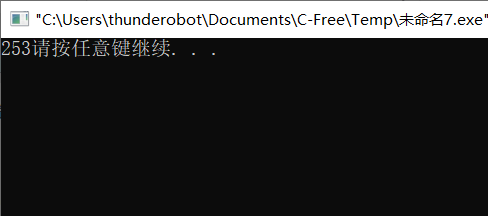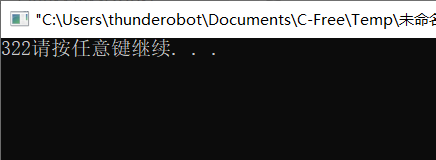• ## vector定义二维数组

千次阅读 2019-09-05 21:49:36
problem: 给定n阶方阵里所有的数，求方阵里...方案一：使用vector #include <iostream> using namespace std; #include <vector> #include <algorithm> int main() { int n,num; int sum=0; ...
problem:
给定n阶方阵里所有的数，求方阵里所有数的和。
solution:
方案一：使用vector
#include <iostream>
using namespace std;
#include <vector>
#include <algorithm>
int main()
{
int n,num;
int sum=0;
cin>>n;
//二维数组的定义
vector<vector<int>> array(n);//行；这里指定了长度，所以每个元素都默认初始化为0了
for(int i=0;i<n;i++)
{
array[i].resize(n);//列
}
for(int i=0;i<n;i++)
{
for(int j=0;j<n;j++)
{
cin>>num;
//array[i].push_back(num);不可以使用这种方式，只是在末尾添加元素
array[i][j]=num;
sum=sum+array[i][j];
}
}
cout<<sum<<endl;
return 0;
}

方案二：使用传统二维数组定义
#include <iostream>
using namespace std;
#include <vector>
#include <algorithm>
int main()
{
int n,num;
int sum=0;
cin>>n;
int array[n][n];
for(int i=0;i<n;i++)
{
for(int j=0;j<n;j++)
{
cin>>num;
array[i][j]=num;
sum+=array[i][j];
}
}
cout<<sum<<endl;
}



展开全文leetcode
• #include<iostream> #include<vector> using namespace std; int main() ... int r = 0, c = 0;...//规定二维数组行数 cin >> r; cout << "Enter c: ";//规定二维数组列数 cin...
#include<iostream>
#include<vector>
using namespace std;

int main()
{
int r = 0, c = 0;
cout << "Enter r: ";//规定二维数组行数
cin >> r;
cout << "Enter c: ";//规定二维数组列数
cin >> c;
vector<vector<int>> array;//定义二维数组
vector<int>v;//定义一维数组
array.clear();//将二维数组清空，或初始化（初始化代码很简单，不会可以百度）//也可不用这一步
int temp = 0;
for (int i = 0; i < r; i++)//输入r*c的二维数组
{
v.clear();//子数组返回时要清除
for (int j = 0; j < c; j++)
{
cin >> temp;
v.push_back(temp);
}
array.push_back(v);
}
for (int i = 0; i < r; i++)//打印输入的二维数组
{
for (int j = 0; j < c; j++)
{
cout << array[i][j] << " ";

}
printf("\n");
}
}


展开全文• 1.利用push_back()实现 #include<...vector> using namespace std; int main() { vector<vector<int> > v; vector<int> a(3,0),b(5,1); v.push_back(a); v.push_back...
1.利用push_back()实现
#include<iostream>
#include<vector>
using namespace std;
int main()
{
vector<vector<int> > v;
vector<int> a(3,0),b(5,1);
v.push_back(a);
v.push_back(b);
cout<<v.size()<<v.size()<<v.size();
return 0;
}可以看到v有两个元素，v即是b，v是a
2.resize实现
#include<iostream>
#include<vector>
using namespace std;
int main()
{
vector<vector<int> > v;
v.resize(3,vector<int> (2,0));
cout<<v.size()<<v.size()<<v.size();
return 0;
}展开全文• 今天在使用vector的二维数组，遇到一个问题。 目的是想使用vector定义一个确定维数的数组，即是数组中每个元素都为一个容器。去网上搜索了一下格式vectorvector > shit （注意string后的尖括号后面哟哟个空格），...
今天在使用vector的二维数组，遇到一个问题。
目的是想使用vector定义一个确定维数的数组，即是数组中每个元素都为一个容器。去网上搜索了一下格式vector<vector<string>
> shit  （注意string后的尖括号后面哟哟个空格），否则被认为格式错误。  而要确定维数 则必须像上述那样vector<vector<string>
> shit(360)    (注意此处是圆括号 不是尖括号)。使用时候如下面的例子
#include <iostream>
#include <vector>
using namespace std;

int main()
{
vector< vector<int> > a(20);
for(int i = 0; i < 20; i++)
{
for(int j = 0; j < 20; j++)
{
a[i].push_back(i*j);
}
}
for(int i = 0; i < 20; i++)
{
for(int j = 0; j < 20; j++)
{
cout  << a[i][j] << " " ;
}
cout << endl;
}
return 0
而在类中定义如此的成员函数的时候，此格式实际上已经初始化了，因此 并不能把此格式应用到类的成员函数中，突发解决方法：
首先定义
class Cxx
{
public：
vector<vector<string> > shit
}

Cxx::Cxx():shit(360)
{
……
}

问题解决~


展开全文• 加入定义一个3*3的数组,数组元素是int类型的 vector> arr(3);//这里的3表示数组有3行 for(int i=0;i { arr[i].resize(3);//这里的3表示每一行有三个元素，即三列 }
• vector> using namespace std; class CMatrixInit { public: CMatrixInit(void); ~CMatrixInit(void); CMatrixInit(int m,int n); bool WriteMatrix(float **arr); public: int m_mValue; int ...
• 就想问一下，vector定义二维数组之后，如何在嵌套的vector容器里面push元素。 注意，是扩充子容器元素。如果定义一个中间容器作为过渡，就要删除原来的行再加入新行，题目要求是不能更改顺序的。代码如下，Arr[i]....
• 如果是普通的定义二维数组 int **p; p = new int*; //注意，int*表示一个有10个元素的指针数组 for (int i = 0; i < 10; ++i) { p[i] = new int; } 定义一个二维整形数组并初始化 如下是定义一个...
• 1 vector二维数组的创建和初始化 vector vec(10,90); //将10个一维动态数组初始为90 vector<vector > vec(row,vector(col,0)); //初始化row * col二维动态数组，初始化值为0 2 获取一维数组的长度 int size = ...数据结构 c++
• vector本来就是可以用来代替一维数组的，vector提供了operator[]函数，可以像数组...1、定义二维vector vector<vector<int> > v;//注意>和>之间的空格。（c++11之后不用注意vector<vecto...
• 维数组定义方法： #include &lt;iostream&gt; #include &lt;vector&gt; using namespace std; int main() { vector&lt;int&gt; arr(10); //arr的长度为10 cout&lt;&lt;arr....
• //定义了一个二维数组，第一维为3，第二维不定长 a.push_back(1);//可直接压入值 cout<<a;//用二维形式调用 vector+vectorvector<vector<int> > a;//定义了一个二维数组，第一维不定...
• int n,m; scanf("%d %d",&amp;n,&amp;m); vector&lt;vector &gt;a[n]; for (int i = 0;i&lt;n;++i) { a[i].resize(m); //定义了一个n*m数组 }
• （1） vector<int> a(10); //定义了10个整型元素的向量（尖括号中为元素类型名，它可以是任何合法的数据类型），但没有给出初值，其值是不确定的。 （2）vector<int> a(10,1); //定义了10个整型元素的...
• Demo #include <... // 定义2*3 二维数组 // vector<vector<int> > a(row, vector<int>(col, 0)); 初始化为0 vector<vector<int> > a(2, vector<int>(3, 0))c++
• ## vector动态二维数组

千次阅读 2008-08-23 17:00:00
定义二维数组 vector > array;(注意后面空一格)有下面几种赋值方式1、 typedef struct tagVector{}vector arr; 2、for(int i=0;i!=10;++i){ vec.push_back(vector (10)); for(int j=0;j!struct
• vector<vector<... 定义一个5行3列的int数组 之后可以在这之上push_back()来增加空间 vector<vector<int> > v1(3); 定义一个3行3列的int数组 for(int i=0;i<3;i++) v1[i]....
• 定义 定义方法一：vector<...定义方法vector<vector<int>>array; 不指定行数 遍历（按方法一） #include<iostream> #include<vector> using namespace std; int main() { in...
• 之前我们分享了STL的一些容器，再介绍vector中只介绍了二维vector定义并没有说二维vector怎么遍历，那么我们今天就来看下二维vector怎么遍历 看下面的代码吧。 #include<stdio.h> //时间复杂度为n...
• c++定义动态二维数组二维指针vector 推荐使用 vector<vector<int>> array(m, vector<int>n);//定义m行n列二维数组 二维指针 二维数组本身相当于是一维数组下存储指针,可以用new int[m]定义一维...
• 因为我自己想在C++方向发展，所以尽可能地用C++提供的STL来完成编程，但是在使用二维数组的时候遇到了麻烦，就是如果用int[][]这种直接表示方式很简单，如果用vector的话就有点麻烦，为此我也查了一下资料。...
• 所以最好在vector二维数组定义时就分配好空间 vector<vector<int>> vec(m, vector<int>(n, 0));//初始化一个m行n列的元素值全为0的二维数组 参考资料： https://www.cnblogs.com/ranzhong/pc++
• #include <iostream> #include <vector> using namespace std; int main() { int row, column; cin >> row >> column; //key code:申请空间 vector<vector&...
• //与sort一起写在同一个函数里面 void do_sth(vector<vector<int>...可避免直接复制数组，提高编译效率 auto cmp = [](vector<int>& a, vector<int>&b） { } ...
• // // //方法1，定义二位列表，初始值都是1，每个位置按照索引，修改值 // class Solution { // public: // vector<vector<int>> generate(int numRows) { // // vector<vector<int>> out; ...
• #include<vector> using namespace std; vector<vector<int>>a;... //添加一维数组 if(i>=a.size())a.push_back(vector<int> {}); a[i].push_back(b[i][j]); ...算法
• C++定义二维数组时候，用vector会初始化为0 int main() { int a; cout<<a<<endl;//会输出地址 vector<vector<int>> a1(2,vector<int>(2)); cout<<a1<......